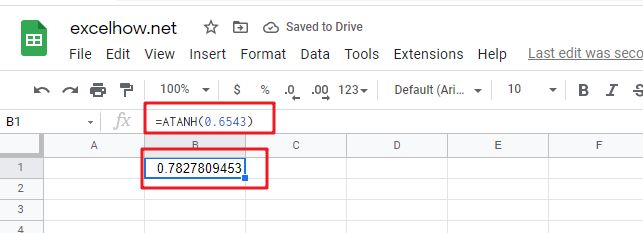This post will guide you how to use Google Sheets ATANH function with syntax and examples.

## Description

The Google Sheets ATANH function returns the inverse hyperbolic tangent of a given number. So you can use the ATANH function to calculate the inverse hyperbolic tangent for a number. The returned result is a numeric value.

The ATANH function is a build-in function in Google Sheets and it is categorized as a MATH function.

## Syntax

The syntax of the ATANH function is as below:

= ATANH (number)

Where the ATANH function arguments is:

• number -This is a required argument. A real number between 1 and -1, but it should exclude the values of -1 and 1.

Note:

• If the number argument is greater than or equal to 1, the ATANH function will return #NUM! Error. If the number argument is less than or equal to -1, the ATANH function will return #NUM! Error.
• If the supplied number is not a numeric value, the ATANH function will get the #VALUE！ Error。

## Google Sheets ATANH Function Examples

The below examples will show you how to use google sheets ATANH Function to return the arctangent of x and y coordinates.

1# get inverse hyperbolic tangent of 0.6543, enter the following formula in Cell B1.

=ATANH(0.6543)

The returned result is 0.782781.Related Posts

This post will guide you how to use Google Sheets LN function with syntax and examples. Description The Google Sheets LN function returns the natural logarithm of a given number.  And the natural logarithms are based on the constant e ...

This post will guide you how to use Google Sheets INT function with syntax and examples. Description The Google Sheets INT function returns the integer portion of a given number. And it will round a given number down to the ...

This post will guide you how to use Google Sheets FLOOR function with syntax and examples. Description The Google Sheets FLOOR function returns a number rounded down to the nearest multiple of significance. So it will return a rounded number. ...

This post will guide you how to use Google Sheets EXP function with syntax and examples. Description The Google Sheets EXP function returns e raised to the power of a number.  The constant e is equal to 2.71828182845904, and it ...

This post will guide you how to use Google Sheets FACT function with syntax and examples. Description The Google Sheets FACT function returns the factorial of a given number.  The factorial of a given number is equal to 1*2*3…* number. ...

This post will guide you how to use Google Sheets EVEN function with syntax and examples. Description The Google Sheets EVEN function rounds a given number up to the nearest even integer. You can use the EVEN function to return ...

This post will guide you how to use Google Sheets COUNTBLANK function with syntax and examples. Description The Google Sheets COUNTBLANK function use to count the number of empty cells in a range of cells. You can use the COUNTBLANK ...